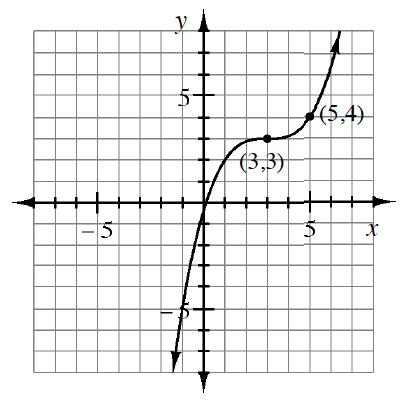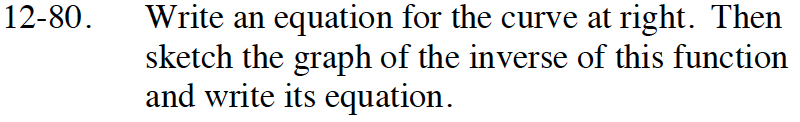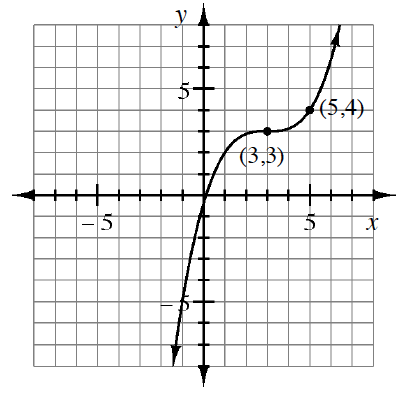Home > CCA2 > Chapter 12 > Lesson 12.1.4 > Problem12-80

12-80.Write an equation for the curve at right. Then sketch the graph of the inverse of this graph and write its equation. Homework Help ✎What parent graph is this similar to?
How is this graph transformed from the parent graph?
Notice that you are given a point other than the locator point so you can solve for a.To find the inverse equation, interchange x and y and solve for y.
To graph the inverse equation, choose some points on this graph and interchange x and y.